## Linear Methods of Applied Mathematics Evans M. Harrell II and James V. Herod*

version of 30 August 1996

Further discussion of the Legendre polynomials and spherical harmonics

While Maple or Mathematica can take care of doing calculations with special functions like the Legendre polynomials, it is helpful to remember where they came from, in order to be able to set calculations up properly. In problems like the one we began with, these special functions occur in the combination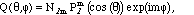where the N's are normalization factors which we may choose at our convenience. Because these come from a self-adjoint eigenvalue problem, they form an orthogonal set, with respect to integration over the angular variables, so the N's can be chosen to make them orthonormal. The result is called a spherical harmonic and denoted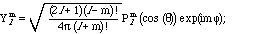(11.8)

it is known to Mathematica, of course, as SphericalHarmonicY[l,m,theta,phi] .

The spherical harmonics are eigenfunctions of the angular part of the Laplace operator, known to physicists as the angular momentum operator: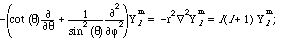(11.9)

Notice that the eigenvalue does not depend on m, but m does show up in one way, because the ranges of the indices are

l = 0, 1, 2, ...; -l <= m <= l .

For each eigenvalue there are 2l + 1 different eigenfunctions, corresponding to the number of values of m between -l and l .

Because the solid angle element on the sphere is sin(theta) d theta d phi, the orthonormality relationship is: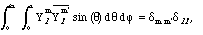(11.10)

which is 0 unless both m=m' and l = l' . Don't overlook the complex conjugation of the second spherical harmonic, which amounts to changing its exp(imphi) to exp(-i m phi). If we rewrite (11.9) in terms of the associated Legendre polynomials and the variable z - notice that sin(theta) d theta = - dz - we find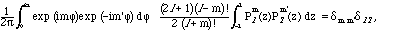which incidentally shows what the orthogonality relationship is for the associated (and usual) Legendre polynomials: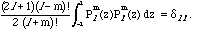(11.11)